Next: Parallel Implementation using Charm++ Up: Insertion Region Selection Previous: Bounding Box Approach   Contents

### Stress-based Selection Approach

A better method for selecting sites for cohesive element insertion is based on a local stress criterion. In this method, an edge is selected for insertion if the some measure of the stresses in the neighboring volumetric elements reaches some critical value. This stress measure depends on the type of failure model characterizing the material of interest. For brittle systems, the stress measure would be based on the value of the maximum principal stress. For more ductile systems, other stress measures based, for example, on the value of the von Mises stress, are more adequate. In the present study, we use the following simple expression of the stress criterion: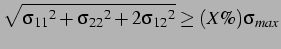(2.34)

where,and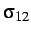are the stresses computed in the adjacent volumetric elements,is a user-defined fraction level and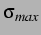denotes the strength of the material.

With this method, cohesive elements are inserted into the system only wherever they are needed, thereby significantly minimizing the computational time and memory costs. To illustrate the concept of stress-based cohesive element insertion, Figure 2.24 presents the result of a dynamic fracture simulation involving a simple L-shaped domain attached along its top edge and subjected to a downward velocity along the left end. In this particular problem, cohesive elements were introduced in the domain when the stress level reached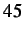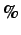of the material strength. No cohesive elements were present in the domain at the beginning of the simulation. The inserted cohesive elements are shown as darker segments and only represent a small portion of the total number of edges, thereby significantly reducing the computational cost. The reference case, with cohesive element present everywhere in the system, took approximately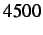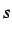, while the simulation based on the stress-based insertion only required about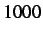of CPU time.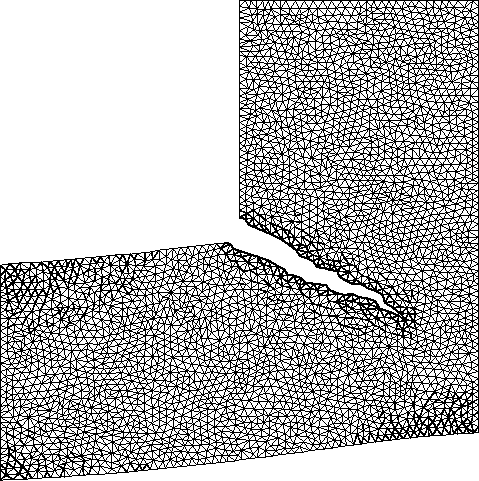Next: Parallel Implementation using Charm++ Up: Insertion Region Selection Previous: Bounding Box Approach   Contents
Mariusz Zaczek 2002-10-13